# KSEEB SSLC Class 10 Maths Solutions Chapter 3 Pair of Linear Equations in Two Variables Ex 3.6

In this chapter, we provide KSEEB SSLC Class 10 Maths Solutions Chapter 3 Pair of Linear Equations in Two Variables Ex 3.6 for English medium students, Which will very helpful for every student in their exams. Students can download the latest KSEEB SSLC Class 10 Maths Solutions Chapter 3 Pair of Linear Equations in Two Variables Ex 3.6 pdf, free KSEEB SSLC Class 10 Maths Solutions Chapter 3 Pair of Linear Equations in Two Variables Ex 3.6 book pdf download. Now you will get step by step solution to each question.

### Karnataka State Syllabus Class 10 Maths Chapter 3 Pair of Linear Equations in Two Variables Ex 3.6

Question 1.
Solve the following pairs of equations by reducing them to a pair of linear equations :
(i) 12x+13y=2
13x+12y=136
(ii) 2x√+3y√=2
4x√+9y√=−1
(iii) 4x+3y=14
3x−4y=23
(iv) 5x−1+1y−2=2
6x−1−3y−2=1
(v) 7x−2yxy=5
8x+7yxy=15
(vi) 6x + 3y = 6xy
2x + 4y = 5xy
(vii) 10x+y+2x−y=4
15x+y+5x−y=−2
(viii) 13x+y+13x−y=34
12(3x+y)−12(3x−y)=−18
Solution:
(i) 12x+13y=2 ……………. (i)
13x+12y=136 ……………… (ii)
From eqn. (i),
12x+13y=2
3y+2x6xy=2
3y + 2x = 12xy
2x + 3y – 12xy = 0
2x + 3y = 12xy …………….. (i)
From eqn. (ii),
13x+12y=136
2y+3x6xy=136
6(2y + 3x) = 13 × 6xy
12y + 18x = 78xy
18x + 12y – 78xy = 0
18x + 12y = 78xy ……………… (ii)
Multiplying eqn. (i) by 4,
4(2x + 3y) = 4 × 12xy
8x + 12y = 78xy …………….. (iii)
From eqn. (ii) – eqn. (iii),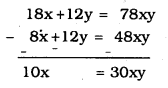x=30xy10=3xy
xx=3y
1 = 3y
y=13
2x + 3y = 12xy
2x+3(13)=12×x×13
2x + 1 = 4x
2x – 4x = 1
-2x = -1
2x = 1
x=12
x=12,y=13

(ii) 2x√+3y√=2 ………….. (i)
4x√+9y√=−1 ………….. (ii)
From eqn (i)
2x√+3y√=2
2y√+3x√xy√=2
3x−−√+2xy−−√=2xy−−√ …………… (i)
From eqn (ii),
4x√+9y√=−1
4y√−9x√xy√=−1
−9x−−√+4y√=−xy−−√
9x−−√−3y√=xy−−√ ……………….. (ii)
Multiplying eqn (i) with 2
2(3x−−√+2y√)=2×2xy−−√
6x−−√+4y√=4xy−−√ ……………….. (iii)
By adding eqn (ii) + eqn (iii)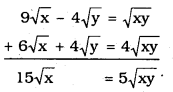x−−√=5xy√15
x√x√=5y√15
1=y√3
3=y√y√=3
∴ y = 9
Substituting the value of ‘y’ in eqn (i)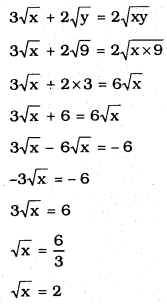∴ x = 4, y = 9

(iii) 4x+3y=14
3x−4y=23
by putting the value of By multiplying eqn. (i) by 4, multiplying eqn. (ii) by 3, and then adding them,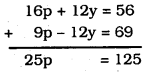∴ p = 5
1x=p=5x=15
Substituting the value of ‘p’ in eqn. (i)
4p + 3y = 14
4 × 5 + 3y = 14
20 + 3y = 14
3y = 14 – 20
3y = -6
∴ y = -2
∴ x⋅=15,y=−2

(iv) 5x−1+1y−2=2
6x−1−3y−2=1
Let 1x−1=p and 1y−2=q
5(1x−1)+1y−2=2 ……………. (i)
6(1x−1)−3(1y−2)=1 …………… (ii)
5p + 1 = 2 …………… (iii)
6p – 3q = 1 …………… (iv)
By solving equation p=13,q=13
Substituting 1x−1 for p,
p=1x−1=13
x – 1 = 3
∴ x = 4
Substituting 1y−2 for q,
q=1y−2=13
y – 2 = 3
∴ y = 5
∴ x = 4, y = 5

(v) 7x−2yxy=5 ……………. (i)
8x+7yxy=15 ………………… (ii)
From eqn. (i)
7x−2yxy=5
7x – 2y = 5xy ………….. (i)
From eqn. (ii)
8x+7yxy=15
8x + 7y = 15xy ……………… (ii)
Eqm (i) × 7 and Eqn. (ii) × 2, we have
49x – 14y = 35xy ………….. (iii)
16x + 14y = 30xy ……………. (iv)
From eqn. (iii) + eqn. (iv)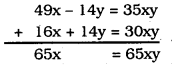∴ 65x65x=y
1 = y
∴ y = 1
Substituting the value of ‘y’ in eqn. (i)
7x – 2y = 5xy
7x – 2 (1) = 5 × x × 1
7x – 2 = 5x
7x – 5x = 2
2x = 2
∴ x = 1
∴ x = 1, y = 1

(vi) 6x + 3y = 6xy ………………. (i)
2x + 4y = 5xy ………………… (ii)
Multiplying eqn. (ii) by 3
3(2x + 4y = 5xy)
6x + 12y = 18xy ………………. (iii)
From eqn. (i) – eqn. (ii)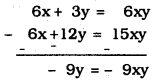9y = 9xy
9y9y=x
1 = x
∴ x = 1
Substituting the value of ‘x’ in eqn (i),
6x + 3y = 6xy
6 × 1 + 3y = 6 × 1 × y
6 + 3y = 6y
6y – 3y = 6
3y = 6
∴ y=63
∴ y = 2
∴ x = 1, y = 2

(viii) 10x+y+2x−y=4
15x+y+5x−y=−2
Let u=1x+y,v=1x−y then,
10u + 2v = 4 …………. (i)
15u – 5v = -2 …………. (ii)
Multiplying eqn. (i) by 5 and multiplying eqn. (ii) by 2 and then by adding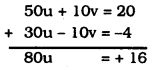∴ u=15
Substituting the value of ‘u’ in eqn. (i)
10×15+2v=4
2 + 2v = 4
2v = 4 – 2
2v = 2
∴ v = 1
∴ u = 1/5 , v =1
1x+y=151x−y=1
∴ x + y = 5 x – y = 1
x + y = 5 …………….. (iii)
x – y = 1 …………….. (iv)
From eqn. (iii) + eqn. (iv)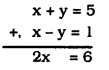x = 3
∴ Substituting the value of ‘x’ in (iii)
x + y = 5
3 + y = 5
y = 5 – 3
y = 2
∴ x = 3, y = 2

(viii) 13x+y+13x−y=34 ………….. (i)
12(3x+y)−12(3x−y)=−18 ……………. (ii)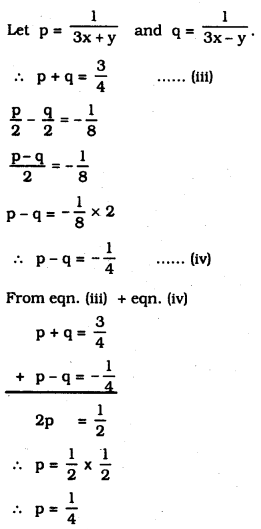Substituting the value of ‘p’ in eqn. (iii)
p+q=34
14+q=34
∴ q=34−14
∴ q=12
∴ p=13x+y=14
3x + y = 4 ……………. (v)
q=13x−y=12
3x – y = 2 ……………… (vi)
From eqn. (v) + eqn. (vi)
3x + y = 4
+3x – y = 2
6x = 6
∴ x=66=1
Substituting the value of ‘x’ in eqn. (v)
3x + y = 4
3 × 1 + y = 4
3 + y = 4
∴ y = 4 – 3
∴ y = 1
∴ x = 1, y = 1

Question 2.
Formulate the following problems as a pair of equations, and hence find their solutions :
(i) Ritu can row downstream 20 km in 2 hours, and upstream 4 km in 2 hours. Find her speed of rowing in still water and the speed of the current.
Solution:
Let speed of Ritu
in still water be x km/hr.
in current water be y km/hr
Speed of water while
upstream is (x + y) km/hr,
downstream is (x – y) km/hr,
2(x + y) = 20
x + y = 10 ………… (i)
2(x – y) = 4
x – y = 2 …………… (ii)
By Adding eqn. (i) and eqn. (ii),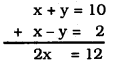∴ x=122=6
Substituting the value, x = 6 in eqn. (i),
x + y = 10
6 + y = 10
y = 10 – 6
∴ y = 4
∴ Speed of Ritu
in still water, x = 6 km/hr.
in current water, y = 4 km/hr.

(ii) 2 women and 5 men can together finish an embroidery work in 4 days,, while 3 women and 6 men can finish it in 3 days. Find the time taken by 1 woman alone to finish the work, and also that taken by 1 man alone. ‘
Solution:
Number of days required
for women be ’x’
for men be ‘y’
Work done by woman in 1 day =1x
Work done by man in 1 day =1y
4(2x+54)=1
2x+54=14 ………….. (i)
3(3x+6y)=1
3x+6y=13 ……………… (ii)
Let 1x=p and 1y=q
∴ 2p+5q=14
8p + 20q = 1 ……….. (iii)
3p+6q=13
9p + 18q = 1 ……………. (iv)
By Cross-multiplication
p−20−(−18)=q−9−(−8)=1144−180
p−2=q−1=1−36
If p−2=1−36 then p=−2−36=118
If q−1=1−36 then q=−1−36=136
p=1x=118∴x=18
q=1y=136∴y=36
∴ Number of days taken by one woman to complete work, x = 18
Number of days taken by one man to complete the work, y = 36.

(iii) Roohl travels 300 km to her home partly by train and partly by bus. She takes 4 hours if she travels 60 km by train and the remaining by bus. If she travels 100 km by train and the remaining by bus, she takes 10 minutes longer. Find the speed of the train and the bus separately.
Solution:
Let speed of the train be x’ km/hr. and Speed of the bus is ‘y’ km/hr.
60x+240y=4 …………… (i)
100x+200y=256 …………… (ii)
If 1x=p1y=q then
60p + 240q = 4 ……………… (iii)
100p+200q=256 ……………….. (iv)
Multiplying eqn. (iii) by 10, Mulitplying eqn. (iv) by 6 and then eqn. (iii) – eqn. (iv), we have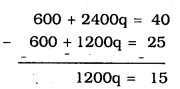∴ q=151200
∴ q=180
Substituting the value of q=180 in eqn. (iii)
60p+240×180=4
60p + 3 = 4
60p =4 – 3
∴ p=160
∴ p=160=1x
∴ x = 60 km/hr.
∴ q=180=1y
∴ y = 80 km/hr.
∴ Speed of train, x = 60 km/hr.
Speed of bus, y = 80 km/hr.

All Chapter KSEEB Solutions For Class 10 Maths

—————————————————————————–

All Subject KSEEB Solutions For Class 10

*************************************************

I think you got complete solutions for this chapter. If You have any queries regarding this chapter, please comment on the below section our subject teacher will answer you. We tried our best to give complete solutions so you got good marks in your exam.

If these solutions have helped you, you can also share kseebsolutionsfor.com to your friends.

Best of Luck!!